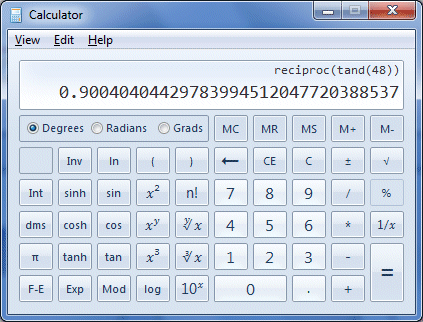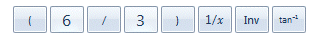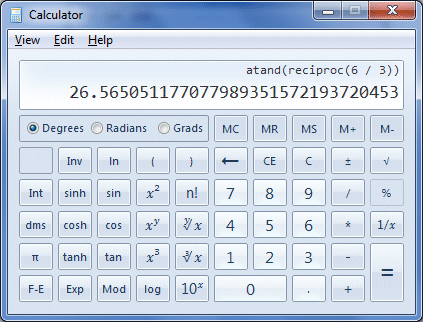# The Cotangent Function

The cotangent function is a trigonometric function, one of three reciprocal functions that we look at in these pages, the other two being the cosecant function and the secant function. A reciprocal function is one that is the reciprocal (or multiplicative inverse) of another function (see below). The cotangent function (usually abbreviated as cot) is the reciprocal function of the tangent function. As with the other trigonometric functions in modern use, the cotangent function was certainly known to Arab scholars, and tables of cotangent values are known to have been in existence by the tenth century CE. In order to clarify exactly how the cotangent function and the tangent function are related, consider the diagram below.The cotangent as a line segment of the unit circle

Like the other trigonometric functions, the cotangent can be represented as a line segment associated with the unit circle. The diagram shows the cotangent for an angle of rotation θ of forty-five degrees (measured anti-clockwise from the positive x-axis). Line segment AF (shown in red) is the cotangent, and lies on the line that is tangent to the circle at point A. Line segment PF is an extension of line segment OP (and, incidentally, also happens to be the secant). Remember that point P is a point on the circumference of the unit circle whose x and y coordinates represent the value of cos (θ ) and sin (θ ) respectively (the line segments representing the sine and cosine are also shown). Remember that one definition of the tangent function is as the quotient of the sine and cosine functions. Click here to see an interactive demonstration that uses the unit circle to show how the sine, cosine and cotangent functions relate to one another (note: your browser must support Java in order for the interactive page to work).

Remember that the tangent function can be defined either as the quotient of the opposite and adjacent, or as the quotient of the sine and cosine functions. If you haven't already deduced the fact from the diagram, you will see from the interactive demo that as the value of sin (θ ) approaches one and the value of cos (θ ) approaches zero, the value of cot (θ ) also approaches zero. As the value of sin (θ ) approaches zero and the value of cos (θ ) approaches one, however, the value of cot (θ ) tends to infinity. The value of cot (θ ) when cos (θ ) equals one is thus said to be undefined. As mentioned, the tangent function is the quotient of the sine and cosine functions. Since the cotangent function is the reciprocal of the tangent function, we can also express the cotangent function in terms of the sine and cosine function:

 cot (θ )   = cos (θ ) sin (θ )

Obviously, since the cotangent function is the reciprocal of the tangent function, it can be expressed in terms of the tangent function as:

 cot (θ )   = 1 tan (θ )

And finally, since the cosine and sine functions are represented by the x and y coordinates respectively of point P (i.e. the point at which the terminal side of angle θ intersects the circumference of the unit circle), we can also conclude that:

 cot (θ )   = x y

We can also define the cotangent function in terms of a right-angled triangle. If you have read the pages in this section covering the three main trigonometric functions (sine, cosine and tangent) you will by now be familiar with the diagram below.In triangle ABC, cot (θ ) = b/a

In right-angled triangle ABC, the tangent of angle θ is the quotient of the opposite and the adjacent (i.e. the sides labelled a and b respectively). Since the cotangent function is the reciprocal of the tangent function, then the cotangent of angle θ will be the quotient of the adjacent and the opposite. This gives us:

 cot (θ )   = cos (θ ) = adjacent = b sin (θ ) opposite a

For those interested in such things, we have used Microsoft Excel to produce our own table of cotangent values for angles ranging from zero degrees () up to three hundred and sixty degrees (360°) in increments of one tenth of a degree. To see the table, click here.

If you study the table, you can see that there is a significant range of angles centred around ninety degrees (90°) and two hundred and seventy degrees (270°) for which the value returned by the cotangent function is quite small and changes relatively slowly. For angles close to zero degrees (), one hundred and eighty degrees (180°) or three hundred and sixty degrees (360°) however, the value returned by the secant function rapidly gets bigger. This is to be expected, since the value of tan (θ ) is very small for these angles, so its reciprocal will be correspondingly large. In fact, the value returned by the cotangent function for an angle of zero degrees, one hundred and eighty degrees or three hundred and sixty degrees is considered to be undefined, since the equation cot (θ ) = 1/tan (θ ) will involve division by zero. In fact, it will apply to any angle for which the tangent value is zero. We present below the graph of the cotangent function for angles in the range zero to seven hundred and twenty degrees (720°).A graph of the cotangent function for angles in the range to 720°

Like the tangent function, the values of the cotangent function will vary between zero and infinity (or more correctly, between zero and a very large undefined value). Because the cotangent function is the reciprocal of the tangent function, the cotangent value will be undefined when the tangent value is zero, and zero when the tangent value is undefined. As you can see by studying the table of cotangent values and the graph of the cotangent function, the cotangent value is negative for all angles for which the sine and cosine have a different sign (i.e. in Quadrant II and Quadrant IV), and positive for all angles for which the sine and cosine have the same sign (i.e. in Quadrant I and Quadrant III).

If you have read the pages on tangents, cosecants and secants (or possibly even if you haven't) you will know that the vertical red lines on the graph are asymptotes. The asymptote of a curve is a line such that as the distance between the curve and the line approaches zero as the value of the curve approaches infinity. In this case, the asymptotes can be considered to be the "gaps" in the graph where the value of cot (θ ) is undefined. Notice also that as the graph crosses the asymptote, the value of cot (θ ) transitions from its maximum positive value to its maximum negative value (or vice versa). You can also see how the values differ on either side of the asymptote by studying the table of cotangent values.

The graph of the cotangent function can include some very large positive and negative peaks, as the value of tan (θ ) tends to zero. Like the tangent function, the period of the cotangent function is one hundred and eighty degrees (180°), or π. You might recall that the tangent function returns a value of zero at zero degrees, and rises to its positive peak as it approaches ninety degrees. It then transitions to its negative peak, returning to zero at one hundred and eighty degrees before repeating the cycle again. The cotangent function is like a mirror image of the tangent function, phase shifted ninety degrees left or right. It falls from its positive peak as it moves away from zero degrees to a value of zero at ninety degrees, and then falls again to its negative peak as it approaches one hundred and eighty degrees. It then transitions to its positive peak and repeats the cycle again.

As with the other trigonometric functions we have looked at, we don't need to use tables to find the cotangent of an angle (or the angle corresponding to a given cotangent value), since any modern scientific calculator can do it for us, even though most calculators don't have a dedicated button for the cotangent function. Finding the cotangent of an angle with the built-in calculator provided in Microsoft Windows is still relatively straightforward. Let's assume we want to find the cotangent of an angle of forty-eight degrees (48°). You can find the Microsoft Windows built-in calculator by clicking on the start button in Windows 7 and selecting All Programs>Accessories>Calculator (other versions of Windows might require a different sequence of keystrokes). The scientific version of the calculator can be selected from the application's View menu. To find the cotangent of forty-eight degrees using the Windows calculator, enter the following keystrokes (if you don't have the Windows calculator, use any available calculator that can perform trig functions):Enter these keystrokes to find the cotangent of 48°

Since the cotangent function is the reciprocal of the tangent function, all we have done here is to use the tangent function button on the calculator, and then apply the calculator's built-in reciprocal function button (usually labelled 1/x) to the result. Make sure, by the way, that you have the calculator set to the Degrees mode (unless you are planning to enter a value for the angle in radians or gradians). If you have entered the keystrokes correctly, you should now see the following display:The calculator displays the value of cot (48°)

So far, so good. Now let's assume that you want to find the size of an acute angle in a right-angled triangle by first finding the quotient of the adjacent and opposite (i.e. the angle's cotangent). Providing you have values for the lengths of the sides of the triangle, you can find the value of the angle's cotangent easily enough. Finding the angle is not quite as straightforward as for the three main trigonometric functions, as most calculators do not have a dedicated button for the arccotangent function (which is the inverse of the cotangent function). Fortunately, there is a relatively simple way around that. Let's find the value of angle θ for the right-angled triangle illustrated below.We want to find the size of angle θ

For angle θ, sides b and a are the adjacent and the opposite respectively. We can actually find the size of the angle in a single operation on the Windows calculator. We do this by using brackets in the key sequence entered, in order to get the calculator to find the inverse of the quotient of the adjacent and opposite (i.e. the cotangent of the angle) first. We then apply the arctangent function to the result. Here is the key sequence to use:Enter these keystrokes to find the size of angle θ

You may have already worked out that what we did here was to find the tangent of the angle by taking the reciprocal of the cotangent. We then simply applied the arctangent function to the result. If you have entered the keystrokes correctly, you should now see a display like the one shown below. If you have read the pages covering the other trigonometric functions, you will by now realise that they are all very closely interrelated. No real surprise then that the graphs of each of the three main trigonometric functions are so similar to the graphs of their respective reciprocal functions. The fact that the trigonometric functions are so closely interrelated means that we can often replace an expression involving one trigonometric function with an equivalent expression involving a different trigonometric function. This can often be very useful.The calculator displays the value of angle θ

The cotangent function, in common with other trigonometric functions, can be represented as an infinite series. The value returned by a trigonometric function for a given angle cannot be calculated using a simple algebraic formula. For most angles, an exact value can only be obtained as the sum of an infinite number of terms, which is obviously impossible to achieve. A reasonably accurate approximation of the value is found using the sum of a limited number of terms from the function's infinite series. The greater the number of terms included, the greater the accuracy of the result. The number of terms used will depend on the accuracy required, and on the computational resources available. The infinite series frequently cited for the cotangent function looks like this:

 cot (x)  = 1 - 1 x  - 1 x3  - 2 x5  - 1 x7  -  · · · · x 3 45 945 4725